Author: Oscar Cronquist Article last updated on August 19, 2019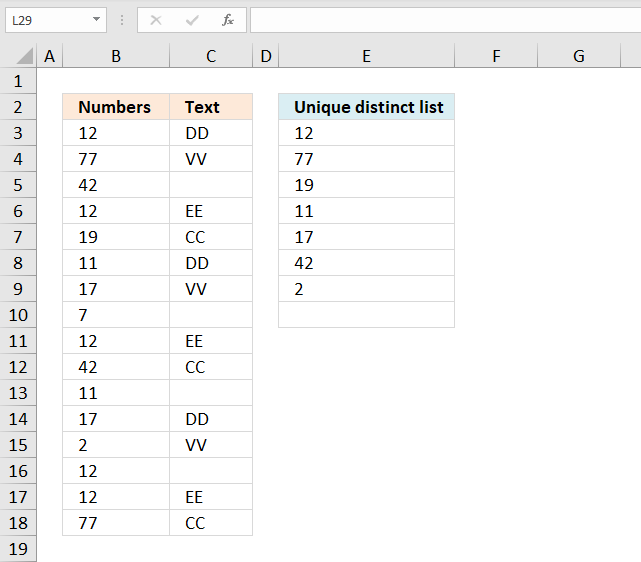Question: I want a unique distinct list to be created from a column where an adjacent column has text cell value on the same row?

Array formula in D3:

=INDEX(\$A\$2:\$A\$17, MATCH(0, COUNTIF(\$D\$1:D1, \$A\$2:\$A\$17)+(ISTEXT(\$B\$2:\$B\$17)=FALSE), 0))

Recommended articles

Recommended articles

VLOOKUP – Return multiple unique distinct values
This article shows how to extract unique distinct values based on a condition applied to an adjacent column using formulas. […]

Recommended articles

Unique distinct list sorted alphabetically based on a condition
This article demonstrates how to extract unique distinct values based on a condition and also sorted from A to z. […]

Recommended articles

Create a unique distinct list based on criteria
The regular formula in cell E6 extracts unique distinct values from column B based on the corresponding number in column […]

How to create an array formula

1. Copy array formula (Ctrl + c)
2. Double press with left mouse button on cell D2
3. Paste array formula (Ctrl + v)
4. Press and hold Ctrl + Shift
5. Press Enter

Recommended article

Recommended articles

A beginners guide to Excel array formulas
Array formulas allows you to do advanced calculations not possible with regular formulas.

#### Explaining formula in cell D3

Step 1 - Check if adjacent values are not text values

The ISTEXT function returns boolean value TRUE if a cell contains a text value and FALSE if not. I am using a cell range as an argument so the function returns an array of bollean values.

They correspond to the input values based on position.

ISTEXT(\$B\$2:\$B\$17)=FALSE

becomes

ISTEXT({"DD";"VV";0;"EE"; "CC";"DD";"VV";0;"EE";"CC";0; "DD";"VV";0;"EE";"CC"})=FALSE

becomes

{TRUE; TRUE;FALSE;TRUE;TRUE; TRUE;TRUE;FALSE;TRUE; TRUE;FALSE;TRUE;TRUE;FALSE;TRUE;TRUE}=FALSE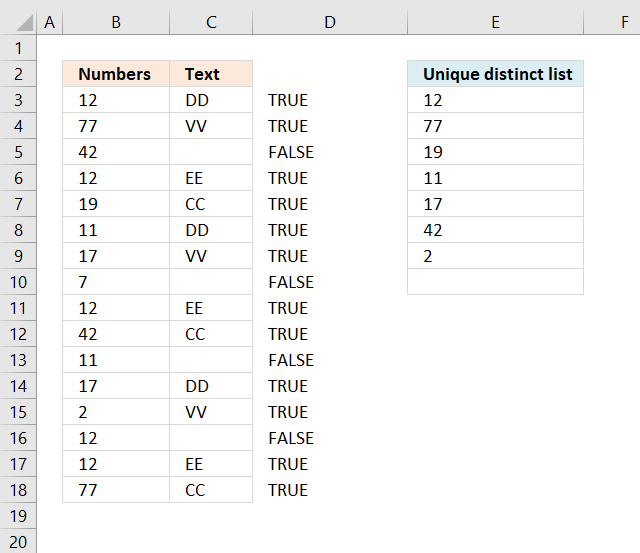We need to know which values are not text values, the array is compared to boolean value FALSE. This will change TRUE to FALSE and FALSE top TRUE, the array becomes:

{FALSE;FALSE; TRUE;FALSE;FALSE; FALSE;FALSE;TRUE;FALSE;FALSE; TRUE;FALSE;FALSE; TRUE;FALSE;FALSE}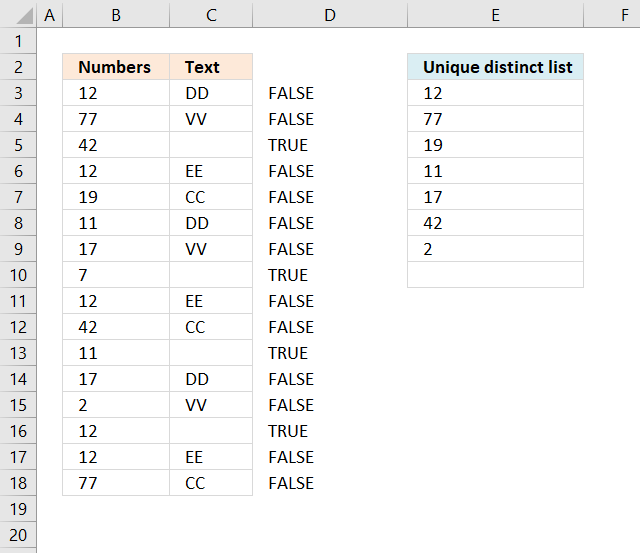Excel handles FALSE as the same as 0 (zero) and TRUE as 1 or more.

Step 2 - Check if value is unique

The COUNTIF function counts values based on a condition or multiple conditions. It has two arguments range and criteria. The first argument is a cell reference that grows when cell is copied to cell below.

It contains a cell reference with two parts, the first one is absolute and the second is a relative cell reference. The COUNTIF function is most often used to return a single value, however, in this case, we need it to return an array of values that match the relative positions of the cell values.

COUNTIF(\$D\$1:D1, \$A\$2:\$A\$17)+(ISTEXT(\$B\$2:\$B\$17)=FALSE)

becomes

COUNTIF(\$D\$1:D1, \$A\$2:\$A\$17)+(ISTEXT(\$B\$2:\$B\$17)=FALSE)

No values have been displayed yet so the array contains 0 (zeros) , if a value had been 1 or more it would have indicated that the value has been displayed in a cell above the current cell.

{0;0;0;0;0;0;0;0;0;0;0;0;0;0;0;0}+(ISTEXT(\$B\$2:\$B\$17)=FALSE)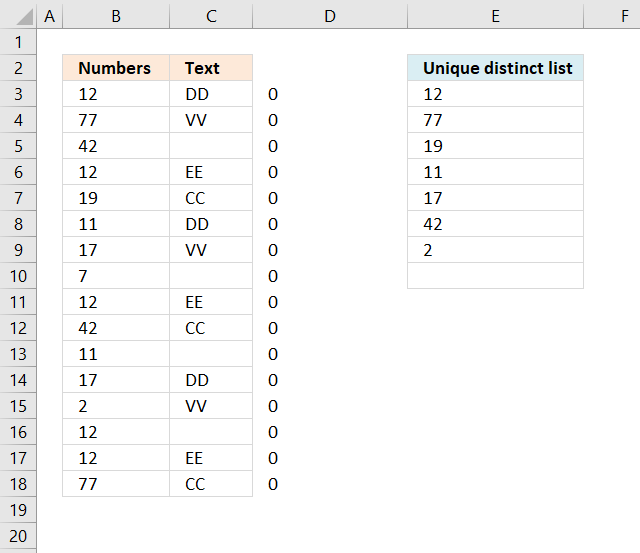becomes

{0;0;0;0;0;0;0;0;0; 0;0;0;0;0;0;0}+{FALSE;FALSE;TRUE; FALSE;FALSE;FALSE;FALSE;TRUE;FALSE; FALSE;TRUE;FALSE;FALSE; TRUE;FALSE;FALSE}

Adding the two arrays is the same as applying OR logic to the arrays meaning 0 + FALSE = 0, 0 + TRUE = 1, 1+FALSE = 1, 1+1 = 2

{0;0;1;0;0;0;0;1;0;0;1;0;0;1;0;0}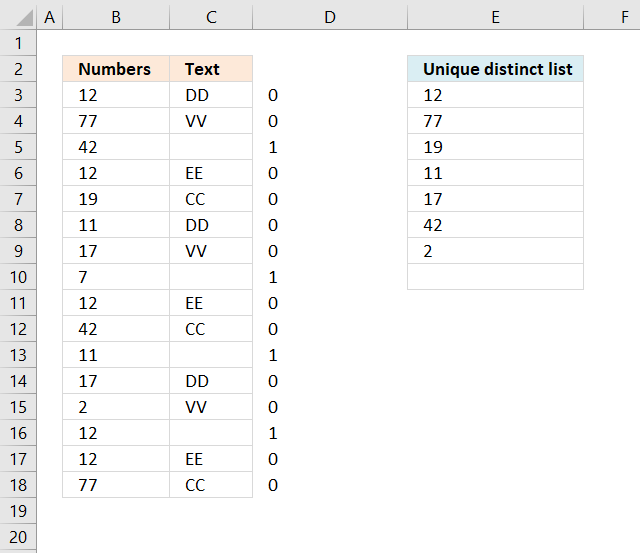This array tells us which values that have not yet been displayed and where the adjacent value is a text value.

Recommended articles

How to use the COUNTIF function
Counts the number of cells that meet a specific condition.

Step 3 - Find relative position

The MATCH function identifies the position of the first cell value that has not yet been shown and where the adjacent value is a text value.

It matches an exact match meaning a value that is exactly equal to 0 (zer0), this is determined by the third argument.

MATCH(0, COUNTIF(\$D\$1:D1, \$A\$2:\$A\$17)+(ISTEXT(\$B\$2:\$B\$17)=FALSE), 0)

becomes

MATCH(0, {0;0;1;0;0;0;0;1;0;0;1;0;0;1;0;0}, 0)

and returns 1.

The MATCH function has identified the first value in the array as a unique distyinct value that has an adjacent cell value that contains a text value.

Recommended articles

How to use the MATCH function
Identify the position of a value in an array.

Step 4 - Return value

The INDEX function simply returns a value from a cell range based on a row and column coordinate. The column coordinate is not neccessary since this example has all values in a single column.

INDEX(\$A\$2:\$A\$17, MATCH(0, COUNTIF(\$D\$1:D1, \$A\$2:\$A\$17)+(ISTEXT(\$B\$2:\$B\$17)=FALSE), 0))

becomes

INDEX(\$A\$2:\$A\$17, 1)

and returns 12 in cell D3.

Recommended articles

How to use the INDEX function
Gets a value in a specific cell range based on a row and column number.

### Get the Excel file